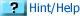You do not need to print this page out. In your notebooks, list the numbered problems and answer the questions next to the appropriate numbers.

 1 Define Newton's 1st Law of motion in a complete sentence.2 Define "inertia" in a complete sentence.3 Watch this YouTube video, http://www.youtube.com/watch?v=wTn9rvBUMK0. There is a bicycle accident at 13 seconds. Describe how this demonstrates Newton's 1st law of motion. 4 Other than adding mass, what can you do to an object to increase it's property of inertia.5 Define Newton's 2nd Law of motion in a complete sentence.6 Define the formula associated with Newton's 2nd law.7 Define each variable an its unit in the formula for Newton's 2nd Law.8 Watch this YouTube video, http://www.youtube.com/watch?v=wTn9rvBUMK0. There is a bicycle accident at 13 seconds. Describe how this demonstrates Newton's 2nd law of motion. 9 A 30 kg child is bouncing on a pogo stick. He accelerates at 29 m/s2 when he bounces upwards. What force did the pogo stick generate to accomplish this task?10 A 50.0 kg bicycle and rider starts from rest and reaches a speed of 10 m/s in 6.00 m. What force did the bicyclist exert to accomplish this task.11 Define Newton's 3rd Law of motion in a complete sentence.12 Watch this YouTube video, http://www.youtube.com/watch?v=wTn9rvBUMK0. There is a bicycle accident at 13 seconds. Describe how this demonstrates Newton's 3rd law of motion.

by Tony Wayne ...(If you are a teacher, please feel free to use these resources in your teaching.)